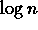Next: Special Numbers and Functions Up: Prime Numbers Previous: What is the current

## Formulae to compute prime numbers

There is no polynomial which gives all the prime numbers. This is a simple exercise to prove.

There is no non-constant polynomial that only takes on prime values. The proof is simple enough that an high school student could probably discover it. See, for example, Ribenboim's book The Book of Prime Number Records.

Note, however, by the work of Jones, Sato, Wada, and Wiens, there is a polynomial in 26 variables such that the set of primes coincides with the set of positive values taken by this polynomial. See Ribenboim, pp. 147-150.

But most people would object to the term ``formula" restricted to mean polynomial. Can we not use summation signs, factorial, and the floor function in our ``formula"? If so, then indeed, there are formulas for the prime numbers. Some of them are listed below.

A reasonable interpretation of the word ``formula" is simply ``Turing machine that halts on all inputs". Under this interpretation, there certainly are halting Turing machines which compute the n-th prime number. However, nobody knows how to compute the n-th prime in time polynomial in. That's still an open question.

Herb Wilf has addressed the question, ``What is a formula?" in his article, ``What is an answer?" which appeared in the American Mathematical Monthly, 89 (1982), 289-292. He draws a distinction between ``formula" and ``good formula". Anyone who claims ``there is no formula for the prime numbers" should read this article.

Here are just a few articles that discuss ``formulas" for primes. Almost all of these do not require computation of the primes ahead of time. Most of them rely on standard mathematical functions such as summation, factorial, greatest integer function, etc.

References

C. Isenkrahe. Math. Annalen 53 (1900), 42-44.

W. H. Mills. Bulletin of the American Mathematical Society 53 (1947), 604.

L. Moser. Mathematics Magazine 23 (1950), 163-164.

E. M. Wright. American Mathematical Monthly 58 (1951), 616-618. (Correction, 59 (1952), 99.)

E. M. Wright. Journal of the London Mathematical Society 29 (1954), 63-71.

B. R. Srinivasan. Journal of the Indian Mathematical Society 25 (1961), 33-39.

C. P. Willans. Mathematics Gazette 48 (1964), 413-415.

V. C. Harris. Nordisk Mat. Tidskr. 17 (1969), 82.

U. Dudley. American Mathematical Monthly 76 (1969), 23-28.

C. Vanden Eynden. American Mathematical Monthly 79 (1972), 625.

S. W. Golomb. American Mathematical Monthly 81 (1974), 752-754.

Algorithmic Number Theory. J.O. Shallit, E. Bach. (to be published, MIT Press).

A Course in Computational Algebraic Number Theory. Henri Cohen. Springer-Verlag, Graduate Texts in Math, 1993.Next: Special Numbers and Functions Up: Prime Numbers Previous: What is the current

Alex Lopez-Ortiz
Mon Feb 23 16:26:48 EST 1998